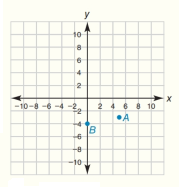Chapter 10.CT, Problem 3CT### Elementary Geometry for College St...

6th Edition
Daniel C. Alexander + 1 other
ISBN: 9781285195698

#### Solutions

Chapter
Section### Elementary Geometry for College St...

6th Edition
Daniel C. Alexander + 1 other
ISBN: 9781285195698
Textbook Problem
1 views

# Use d = ( x 2 - x 1 ) 2 + ( y 2 - y 1 ) 2 to find the length of C D - as described in Exercise 2.                                 _ In the coordinate system for Exercise 1, plot and label each point: C ( - 6 ,   1 ) and D ( 0 ,   9 )To determine

To find:

The length of CD¯ using distance formula where the coordinates are C(-6, 1) and D0, 9.

Explanation

Given points are,

C(-6, 1) and D(0, 9)

Point C is located at a distance from origin in the x-axis =-6

Point C is located at a distance from origin in the y-axis =1

Similarly, point D is located at a distance from origin in the x-axis =0

Point D is located at a distance from origin in the y-axis =9

Sketch the given points in graph and name them as C and D and joined the points as shown in the figure,

Formula for distance is, d=(x2-x1)2+(y2<

### Still sussing out bartleby?

Check out a sample textbook solution.

See a sample solution

#### The Solution to Your Study Problems

Bartleby provides explanations to thousands of textbook problems written by our experts, many with advanced degrees!

Get Started

#### Find more solutions based on key concepts